## Vector Space

A vector space over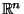is a set of Vectors for which any Vectors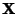,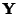, and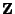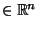and any Scalars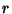,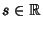have the following properties:

1. Commutativity: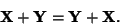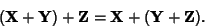3. Additive identity: For all,4. Existence of additive inverse: For any, there exists a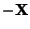such that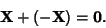5. Associativity of scalar multiplication: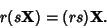6. Distributivity of scalar sums: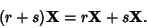7. Distributivity of vector sums: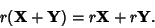8. Scalar multiplication identity: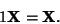An-D vector space of characteristic two has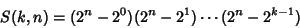distinct Subspaces of Dimension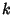.

A Module is abstractly similar to a vector space, but it uses a Ring to define Coefficients instead of the Field used for vector spaces. Modules have Coefficients in much more general algebraic objects.

See also Banach Space, Field, Function Space, Hilbert Space, Inner Product Space, Module, Ring, Topological Vector Space

References

Arfken, G. Mathematical Methods for Physicists, 3rd ed. Orlando, FL: Academic Press, pp. 530-534, 1985.